# Formula Overview

A formula is an operational expression composed of functions, operators, and values.You can use a formula to reference the fields to be calculated and get expected output values.

Formula can help you avoid mistakes resulted from manual calculation, increasing efficiency of data management.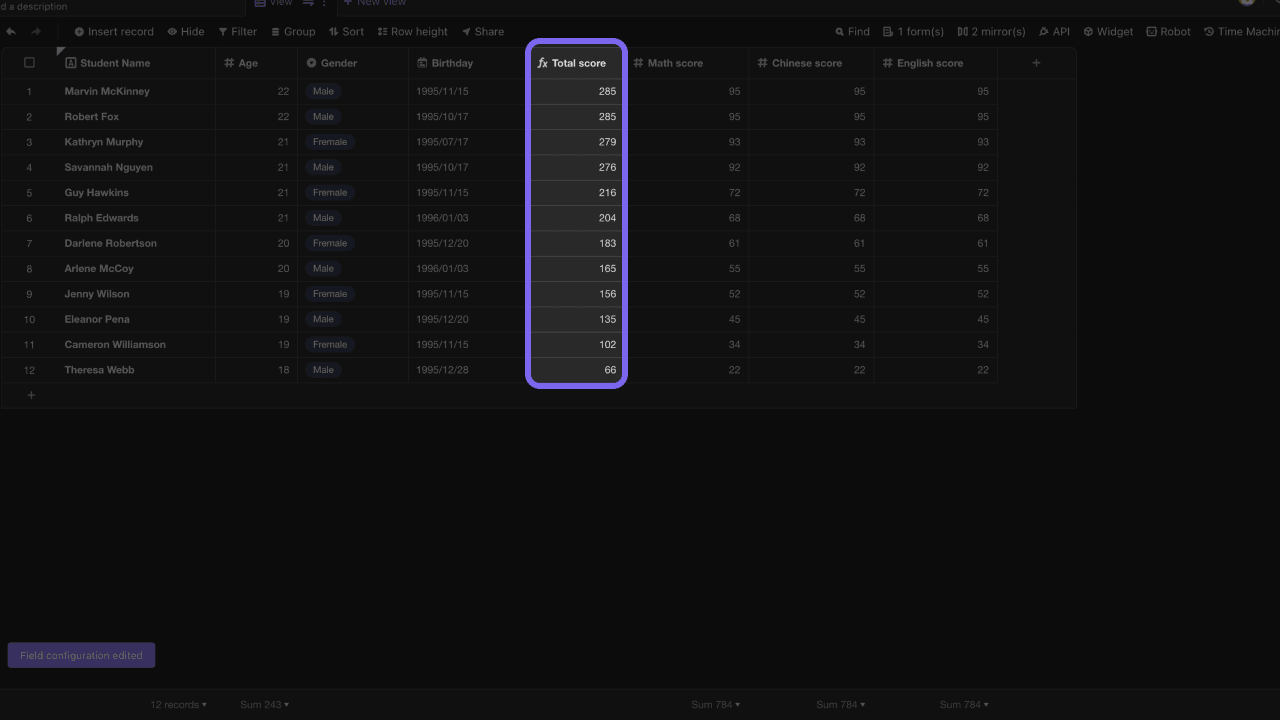## Differences with Excel​

Although AITable's formula is similar to Excel's formula, there is still a distinct difference:

AITable formula field will reference an entire field of data rather than specific cells.

For example, to calculate the total grade of a student, you need to reference the data of three separate cells in Excel. As the figure shows below, the formula is written as: `F2+G2+H2`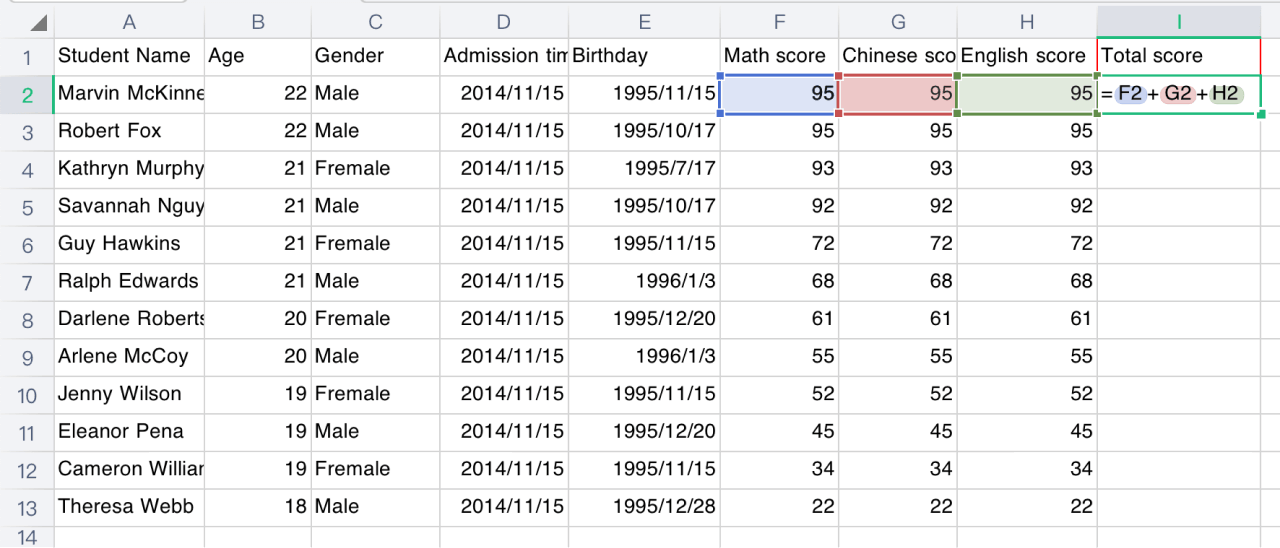However, in AITable, you need to reference the three fields of "Math Score", "Chinese Score" and "English Score", and write the formula as: `{Mathematics score}+{Chinese score}+{English score}`## Quick start​

### How to create a formula​

To create a formula, insert a new field first and select "Formula" field type. Then, click the text box to enter a formula.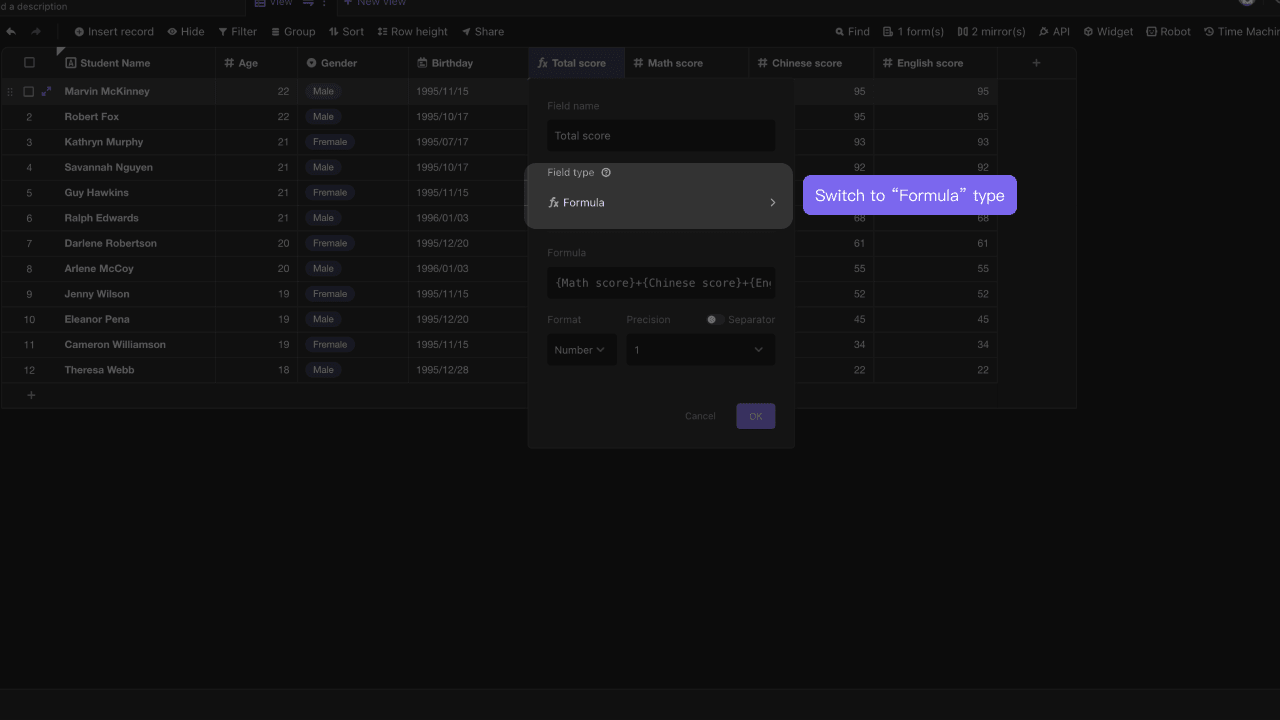### Writing a formula​

The configuration menu of formula field consists of three sections, including a text box for entering formula, a left suggestion list that suggests you the existing fields and functions you can use, and an introduction section showing function description and using examples.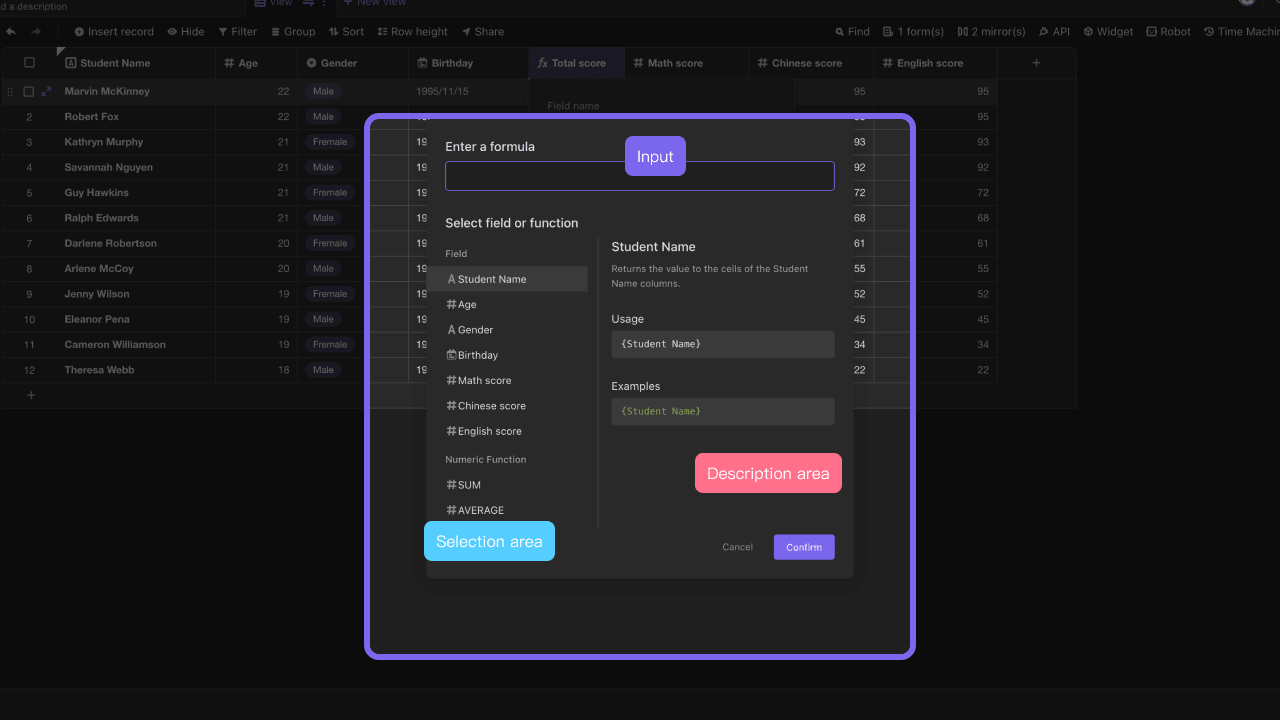Before you start writing formulas, you need to learn the following essentials:

• **Field: an existing field in the current datasheet, which you can reference its name in the formulas. Usually, you need to use curly bracket to reference field names.**

For example, "name" field is referenced as: `{name}`

• **Function: A predefined formula that calculates input values to output values for you.**

SUM is an example function: `SUM({Math score}, {Science score}, {History score})`

• **Operator: A symbol that can perform logical or arithmetic operations.**

Example operators: addition "+", subtraction "-", multiplication "*", division "/"

• **Parameter: any data you want to reference in your formula are parameters.**

For example, in formula of `({Math score} + {Science score} + {History score}) / 3`, {Math score} and "3" are parameters.

For more writing tips please see

Formula Writting Tips

Here are a few more examples to let you see what the formulas could be like:

``({Math score}+{Science score}+{History score})/3{name}&"-"&{age}&"-"&{country of origin}MAX({math score}, {Chinese score}, {English score})IF({average score} > 60, "👍Pass", "❗Fail")``

You've seen a lot of examples, now follow us writing a formula~

At first, insert three number type fields into the datasheet, named as "Math Scor e", "Chinese Score" and "English Score".

Secondly, make up some score data and fill then into the fields created in first step, and then create a new formula field.

The last step, double click formula field header to open configuration menu, and then enter a formula into the text box as:`{math score}+{Chinese score}+{English score}`. After this, click save and exit the field configuration menu, and you can see the operation is automatically executed.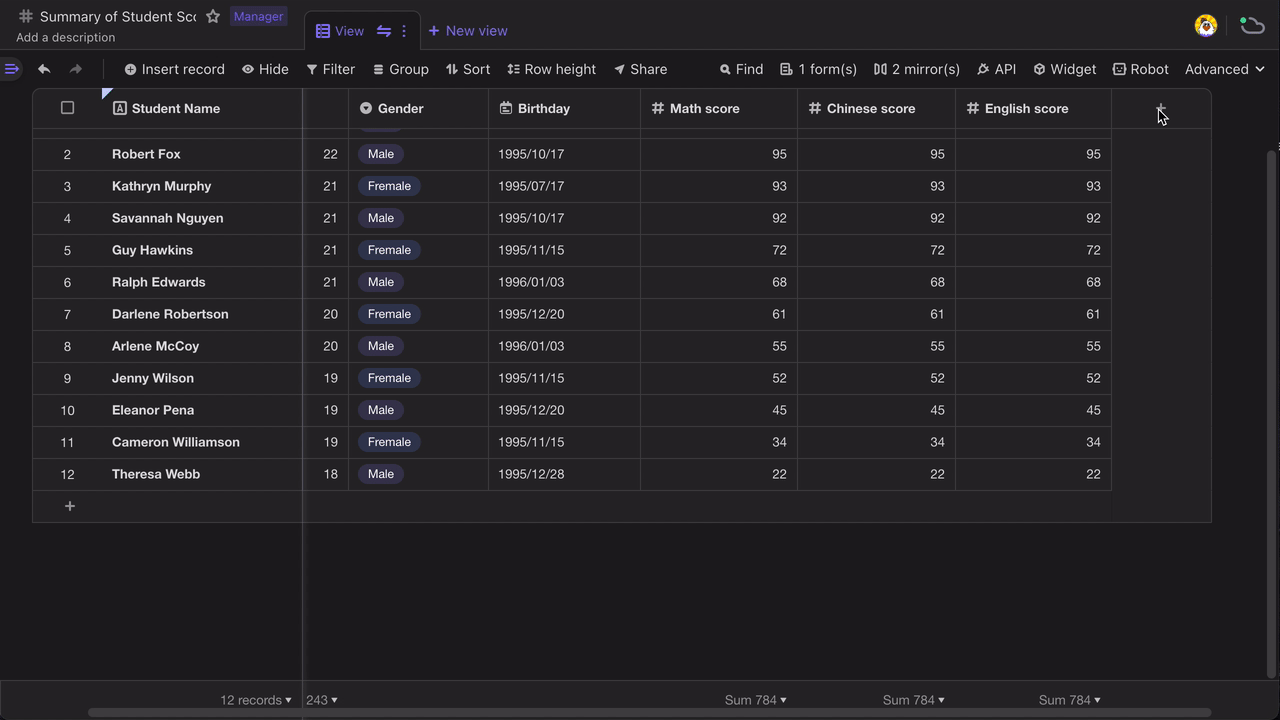Congratulations~ You've successfully learned how to write and use a formula.For more functions and operators, please refer to Summary of Functions and Operators.

### Formatting the output data​

When the output data of a formula is a number or date type, you can reformat it.

For number type

When the output data of the formula is number, you can set decimal places you want to show.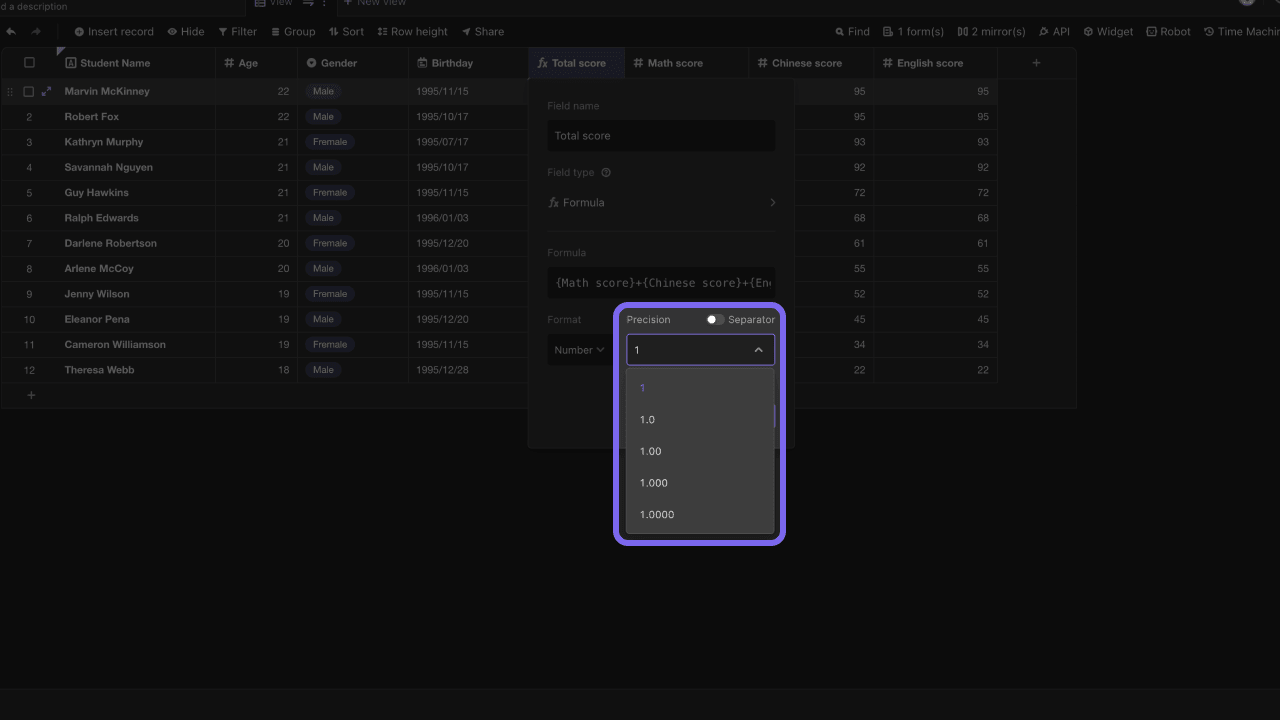For date type

When the output data of the formula is date, you can reformat it and determine whether to display time.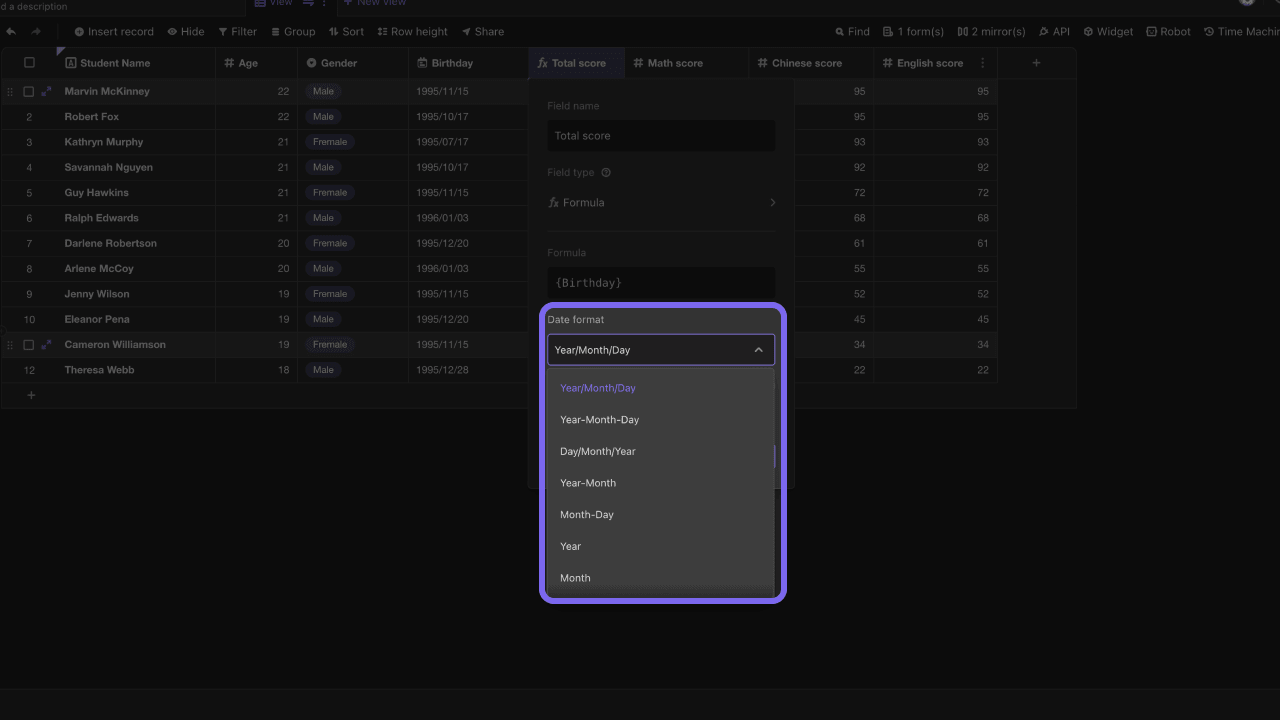To know more about functions and formula writing tips, you can see listed articles below.